# Novel two-dimensional tetrahexagonal boron nitride with a sizable band gap and a sign-tunable Poisson's ratio†

Mehmet Emin Kilic* and Kwang-Ryeol Lee *
Computational Science Center, Korea Institute of Science and Technology, Seoul 136-791, Republic of Korea. E-mail: mekilic@kist.re.kr; krlee@kist.re.kr

Received 3rd February 2021 , Accepted 19th April 2021

First published on 19th April 2021

## Abstract

By performing first-principles calculations, a new two-dimensional (2D) boron nitride (th-BN) with perfectly ordered arrangements of tetragonal and hexagonal rings is predicted to be energetically, dynamically, thermally, and mechanically stable. The unique structure endows th-BN with anisotropic mechanical, electronic, and optical properties. Remarkably, th-BN exhibits exceptional mechanical properties such as high in-plane stiffness and sign-tunable Poisson's ratio (PR). The PR of th-BN gradually decreases with the increase of axial strain and even becomes negative at a very small strain (∼2%), which is novel, thereby offering the ability to become non-auxetic, auxetic, and partially auxetic 2D nanomaterials depending on the strain rate and direction. The structure can withstand tensile strain as large as 36%, and shows ultrahigh ideal strength that can even outperform graphene and hexagonal BN. The th-BN is a natural 2D semiconductor with an indirect wide band gap of 4.49 eV. The band gap can be tuned by applying lattice strain and hydrogenation. The full hydrogenated th-BN exhibits an indirect-to-direct band gap transition. The th-BN shows high optical absorption in the ultraviolet region. The optical absorption spectrum is highly direction-dependent and tunable by strain, suitable for high-performance optoelectronic device applications. Furthermore, th-BN can be stacked into two different configurations, and are dynamically stable and exhibit exotic electronic properties. The desirable direct band gap and anisotropic effective mass of the th-C/th-BN heterostructure suggest that th-BN can be a suitable substrate for tetrahexcarbon.

## 1. Introduction

Nowadays, most theoretical and experimental efforts have been focused on two-dimensional (2D) materials.1–5 Graphene is one of the most promising materials due to its atomic thickness and remarkable properties including extremely high thermal conductivity, high carrier mobility, and extraordinary high mechanical strength and stiffness.6–9 Hexagonal boron nitride (h-BN) has been experimentally synthesized10,11 and exhibits unique properties such as high mechanical hardness, thermo-chemical stability, and good electrical and thermal conductivity.12 Although h-BN is an isoelectric analogue of graphene (having the same geometry and the number of valence electrons), its properties are quite different from graphene (i.e. graphene is metallic with a zero band gap, while h-BN is an insulator with a large band gap). Gannett et al. have reported that h-BN is a promising substrate for the direct growth of graphene on an insulating substrate.13 The strong in-plane bonds, large band gap of about 4.71 eV, and planar structure of h-BN make it an ideal flat, insulating, and inert surface.14–16

To date, considerable attention has been dedicated to 2D materials with hexagonal structures.17 However, the hexagonal form is not the only possible structure for C- or BN-based 2D materials. It is known that an atom prefers a spatial arrangement of multiple bonds with its neighbors due to the valence electron pair repulsion rule.18 This tendency suggests that there should exist some other possible 2D structures consisting of the combination of 4-, 5-, and/or 6-membered rings.19 Recently, a new 2D carbon allotrope, known as tetrahex-carbon (th-C), has been theoretically proposed,20 which is a direct band gap semiconductor with an intrinsic band gap of 2.63 eV (ref. 20 and 21) and has outstanding electronic and mechanical properties such as high carrier mobility,20,22 sign-tunable Poisson's ratio,22 and ultrahigh ideal strength outperforming graphene.21 A great effort has been devoted to improving the mechanical, thermal, electronic, and optical properties of th-C via hydrogenation21 and fluorination.23 For instance, theoretical investigations have revealed that hydrogenation and fluorination can effectively tune the phononic gap and electronic band gap of th-C and induce direct–indirect–direct band gap transitions.21,23 The 2D tetrahexagonal compounds of group-IV atoms (C, Si, Ge and Sn), known as tetrahex carbides, have recently been proposed, possessing robust stability and superior properties such as direct band gap, high anisotropic carrier mobility, and good optical absorption in the visible range, very promising for photocatalytic water splitting applications.22 Very recently, tetrahexagonal aluminum nitride (th-AlN), having exotic mechanical behaviors such as good flexibility, negative Poisson's ratio, and ultrahigh ideal strength, has been predicted as a promising candidate for photocatalytic water splitting.24

Inspired by the versatility of 2D tetrahexagonal semiconductors and their appealing properties for nano- and opto-electronics, we design a stable tetrahexagonal sheet made of B and N atoms, isostructural to th-AlN. Due to the large electronegativity difference between B and N, the tetrahexagonal structured BN sheet (th-BN) can offer modified electronic properties, different from that of th-C. In this paper, first-principles calculations have been used to predict the properties of th-BN. In particular, we have investigated its energetic, dynamic, thermal, and mechanical stability by performing the total energy calculations, phonon calculations, ab initio molecular dynamics (AIMD) simulations, and elastic constant calculations, respectively. Next, we have investigated its mechanical, electronic, and optical properties. We have further shown that the hydrogenated derivatives of th-BN and its bilayer counterparts show interesting electronic properties such as band gap transition and modification. Finally, we have revealed the superior electronic properties of the th-C/th-BN heterostructure.

## 2. Computational methods

Density functional theory (DFT) calculations were carried out within the projector-augmented-wave formalism25,26 using a plane-wave basis set, encoded in the Vienna Ab initio Simulation Package (VASP).27 A plane wave with a cutoff energy of 520 eV was used. The exchange and correlation energies were calculated with the functional of Perdew, Burke, and Ernzerhof (PBE) in the generalized gradient approximation (GGA).28 A vacuum region of 20 Å was added along the out-of-plane direction to ensure that no appreciable interaction occurs between the two adjacent layers. The k-points in the reciprocal space were sampled using the Γ-centered Monkhorst–Pack scheme29 with a 24 × 24 × 1 grid. The atomic relaxation was carried out until the change in the total energy and atomic force components per one unit cell was smaller than 10−5 eV and 10−4 eV Å−1, respectively. The phonon properties were calculated using the PHONOPY code30 based on the density functional perturbation theory (DFPT) method with a 4 × 4 × 1 super cell and a 2 × 2 × 1 k-mesh where the convergence criterion of the total energy was set as 1 × 10−8 eV per unit cell. Moreover, AIMD simulations were performed under the constant volume and temperature (NVT) ensemble, where the temperature was controlled using a Nose–Hoover thermostat.31–33 The AIMD simulations ran for a total simulation time of 6 ps with time steps of 1 fs at 900 K using a 4 × 3 × 1 supercell with a 2 × 2 × 1 k-mesh. For electronic properties, since the PBE functional fails to capture correctly the electronic band gap of the th-BN monolayer, we also employed the Heyd–Scuseria–Ernzerhof hybrid functional (HSE06).34,35 Moreover, the optical response calculations were carried out using the quasi-particle many-body GW0 approximation36 with a cutoff energy of 480 eV. In order to achieve the numerical accuracy in the GW0 approximation, virtual orbitals are chosen 3 times greater than the number of occupied bands. The optical absorption spectra including electron(e)–hole(h) interactions were calculated via the Bethe–Salpeter equation (BSE).37

## 3. Results and discussion

### 3.1. Atomic structure and bonding

The optimized atomic structure and possible formation mechanism of the proposed th-BN sheet are presented in Fig. 1. With the aim of deriving the structure of th-BN, we use 2D tetragonal BN (t-BN) obtained from cubic zinc blende bulk phase as an initial structure. The th-BN structure is locally formed from t-BN upon shifting atoms along the zigzag direction, as shown in Fig. 1(a). Moreover, the structure of th-BN can be perfectly formed by applying the Stone–Wales transformation to the Cairo pentagonal BN (p-BN)38 as depicted in Fig. 1(b). Thus, th-BN can be formed from either t-BN or p-BN. The optimized atomic structure of th-BN is presented in Fig. 1(c). The orthorhombic lattice, composed of tetra- and hexa-rings of B and N atoms, creates a Pccm symmetry (space group no. 49). The unit cell contains 3-fold and 4-fold coordinated atoms (denoted as X3 and X4, X = B, N). We define two buckling thicknesses as h1 = 0.988 Å and h2 = 1.361 Å, where h1 defines the difference of the vertical coordinates between the B3 atoms and h2 specifies this distance between the N3 atoms in the top and bottom layers. Due to the unique atomic structure of th-BN, we compare the properties of tetrahexagonal (th-) BN to those of tetragonal (t-) and hexagonal (h-) BN. As a benchmark, we determined the optimized atomic structure, phonon band dispersions, and electronic and mechanical properties of t-BN (Fig. S1) and investigated the structural parameters and lattice dynamics of h-BN (Fig. S2). The obtained results agree well with the literature.39 We then turn our attention to the structure of th-BN. The optimized lattice constants are found to be a = 4.560 Å and b = 6.203 Å. The calculated bond length for B3–N3 is about d1 = 1.355 Å, which is 0.01 Å shorter than that of h-BN. Moreover, the bond lengths of B3–N4 and B4–N3 are d2 = 1.533 Å and d3 = 1.583 Å, respectively, which are slightly larger than the B–N bond length in t-BN (1.578 Å). The obtained structural parameters of 2D th-BN are given in Table 1.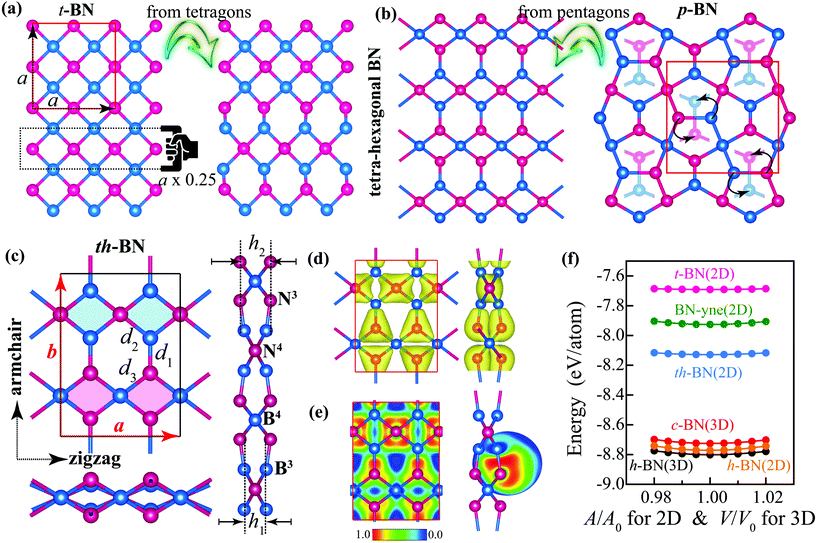Fig. 1 Deriving the structure of th-BN from (a) the tetragonal lattice and (b) the Cairo pentagonal lattice. (c) Top and side views of the optimized atomic structure of th-BN. The unit cell is marked by a black solid rectangle, as shown in the inset. (d) Total charge density distribution, where the value for the isosurface is 0.08 e Å−3. (e) Electron localization function (ELF) contour plot of th-BN, where the color scale for the ELF values is given at the bottom of the figure. The ELF is dimensionless and ranges from 0 (represents a lack of electrons) to 1 (represents highly localized and bound electrons). (f) Area (volume) dependence of total energy per atom for some 2D (3D) BN compounds along with their different forms having the same stoichiometry.
Table 1 Calculated lattice parameters a and b in Å, buckling thicknesses h1 and h2, bond lengths d1, d2, and d3 in Å, and the electronic band gap energy by the PBE functional (EPBEgap) and HSE06 hybrid functional (EHSE06gap) methods in eV, and the band gap type for th-C, th-BN, 4H-th-BN-4H, bil1-th-BN, bil2-th-BN, and th-C/th-BN
a b h 1 h 2 d 1 d 2 d 3 E PBEgap E HSE06gap Type
aRef. 21.
th-Ca 4.533 6.107 1.160 1.160 1.340 1.540 1.540 1.62 2.63 Direct
th-BN 4.560 6.203 0.988 1.361 1.355 1.533 1.583 3.24 4.49 Indirect
4H-th-BN-4H 4.503 6.178 1.646 1.602 1.616 1.549 1.597 3.99 5.15 Direct
bil1-th-BN 4.558 6.193 2.314 1.351 1.529 1.577 2.64 3.79 Direct
bil2-th-BN 4.487 6.178 1.547 1.376 1.569 1.597 4.18 5.55 Indirect
th-C/th-BN 4.538 6.118 2.262 1.342/1.353 1.537/1.524 1.537/1.575 1.27 2.22 Direct

To gain insight into the nature of bonding in th-BN, the Bader charge analysis was performed.40 We found that there are certain electronic charge transfers from the less electronegative boron atoms to more electronegative nitrogen atoms. The charge transfer on the B3, B4, N3, and N4 atoms are −2.35e, −2.45e, 2.32e, and 2.51e, respectively, indicating that there are different charge transfers from the B3 and B4 atoms to the nitrogen atoms. The B4 atoms are losing more electrons than the B3 atoms, revealing that the B4–N3 bond is more ionic and weaker than the B3–N3 bond. This behavior is confirmed by the total charge density distributions of th-BN where the charges are mainly localized around both N atoms and the center of B–N bonds (Fig. 1(d)). To achieve a better insight into the bonding nature of th-BN, we analyzed the electron localization function (ELF)41 for the B3–N3, B3–N4, and B4–N3 bonds (Fig. S3). The ELF bonding analysis depicted in Fig. 1(e) clearly indicates the σ and “half-i” bonds between the B3 and N3 atoms, suggesting that the bonds are in a covalent regime. However, the low electron density around the B3 atoms in the B3–N3 bond can be considered as a feature of partially ionic bonding. Thus, the chemical bonding for the B3–N3 bond is of mixed ionic–covalent nature. Likewise, the B3–N4 and B4–N3 bonds can also be considered to be of ionic–covalent nature.

### 3.2. Energetic stability

To evaluate the energetic stability of th-BN, we performed the total energy calculations and compared the results with other BN materials having the same stoichiometry. Fig. 1(d) shows that th-BN is energetically stable in between h-BN and t-BN, in a way that, the calculated total energy of th-BN (−8.130 eV per atom) is relatively higher than that of h-BN (−8.800 eV per atom), but lower than that of the 2D BN-yne42 (−7.926 eV per atom) and tetragonal BN (t-BN) (−7.691 eV per atom). Thus, th-BN is energetically favorable. We further computed the cohesive energy of th-BN with reference to the spin-polarized B and N atoms. The cohesive energy is defined as Ecoh = (EBNnBEBnNEN)/(nB + nN), where EBN, EB, and EN are the total energy of th-BN, and the total energies of the free B and N atoms, respectively, and nB and nN represent the total number of B and N atoms in the unit cell, respectively. The calculated negative cohesive energy of −6.13 eV per atom for th-BN indicates the strong bonding between the B and N atoms in th-BN. We then discuss the possibility of experimental realization of th-BN by evaluating its formation energy. We define the formation energy as the total energy difference between th-BN and its reference bulk forms as follows: ΔEc/h = E2DEc/h3D, where E2D, Ec3D, and Eh3D are per atom energies of the th-BN sheet, and cubic zinc-blende (c-) and hexagonal (h-) BN as bulk reference materials, respectively. The low energy of ΔEc and ΔEh enables easy fabrication of the 2D th-BN from its reference bulk counterparts of c-BN(3D) and h-BN(3D), respectively. The calculated ΔEc and ΔEh are 596 meV per atom and 672 meV per atom, respectively, which are lower than that of some experimentally synthesized 2D compounds such as graphdiyne (823 meV per atom)43 and silicene (750–800 meV per atom),44,45 indicating the possibility of experimental realization of th-BN on a suitable substrate.46
3.2.1. Dynamic stability. To further examine the dynamic stability of th-BN, we calculated the phonon dispersion curves using ab initio lattice dynamics. The phonon band structure and atom-resolved phonon density of states (PhDOS) are presented in Fig. 2(a). It can be seen that th-BN is dynamically stable as there are no imaginary frequencies throughout the BZ. The PhDOS results show that the high-frequency modes are mainly characterized by the motion of 3-fold coordinated atoms (B3 and N3). Remarkably, the highest phonon frequency of th-BN (1629 cm−1) is higher than that of t-BN (1218 cm−1) (Fig. S1), h-BN (1521 cm−1) (Fig. S2), z-BN (1100 cm−1),47 silicene (580 cm−1),48 MoS2 (470 cm−1),49 and phosphorene (440 cm−1).50 Thus, this high phonon frequency indicates the robustness of the B–N bonds in the th-BN structure. Next, we investigate the dynamic stability of th-BN when subjected to external strain (10% biaxial tensile strain), which is important for practical applications as 2D materials are extremely sensitive to the environment due to their inherent large surface to volume ratios. Fig. 2(b) shows the calculated phonon band dispersions of the strained th-BN, where all the vibration modes are still positive. Therefore, th-BN maintains its dynamic stability even under strain.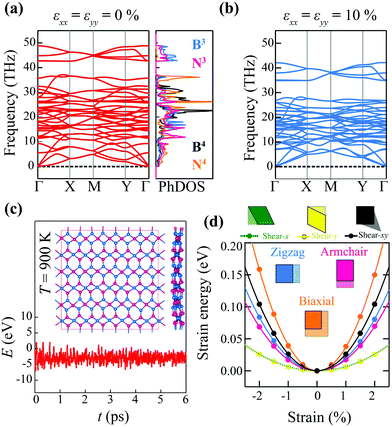Fig. 2 (a) Calculated phonon dispersions and phonon density of states (PhDOS) of th-BN and (b) phonon band dispersions of 10% tensile strained th-BN. The band dispersions are plotted along the path Γ(0, 0, 0) → X(1/2, 0, 0) → M(1/2, 1/2, 0) → Y(0, 1/2, 0) → Γ(0, 0, 0). (c) Variation of the total energy calculated by AIMD simulations at 900 K with respect to time. In the inset is shown the snapshot of the atomic configuration after 6 ps. (d) Calculated strain energy with respect to uniaxial strains along the zigzag and armchair directions, biaxial strain, and various in-plane shear strains (shear-x, shear-y, and shear-xy), as shown in the inset.
3.2.2. Thermal stability. A 2D material needs to be stable under ambient conditions to become applicable in conventional devices. To make sure that th-BN is stable under these conditions, we performed AIMD simulations at a high temperature (900 K) for 6 ps. As the energy is sensitive to the bond length and bond angle changes, we monitor and analyze the total energy of the system. Fig. 2(c) shows that the total energy of th-BN fluctuates around a certain energy value during the simulation time, indicating a structural equilibrium at 900 K. Moreover, the atomic structure in the AIMD snapshot at the end of the simulation time verifies no bond breaking or bond reforming. Therefore, even at high temperatures, the structural integrity is well maintained as the initial structure, confirming the thermal stability of th-BN.
3.2.3. Mechanical stability. To discuss the mechanical stability of th-BN, we calculated the in-plane elastic constants. The orthorhombic structure of th-BN possesses nine independent second-order elastic constants (C11, C12, C13, C22, C23, C33, C44, C55, and C66). The mechanical stability is evaluated by Born's stability criteria as51C11C22C122 > 0 and C66 > 0. We calculate the corresponding elastic constants by fitting the curve of the energy–strain relationship. The equation is given below: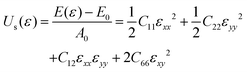(1)
where Us(ε) is the strain energy per area (A0), E(ε) and E0 are the system's total energies with and without strain (εi), respectively. The variation of the strain energy of th-BN with respect to zigzag, armchair, biaxial, and shear strains is presented in Fig. 2(d). The obtained elastic constants C11 = 232.46 N m−1, C22 = 195.49 N m−1, C12 = 4.17 N m−1, and C66 = 73.41 N m−1 meet the elastic stability criteria for th-BN.

### 3.3. Mechanical properties

To verify the robust stability of th-BN, we investigated its mechanical properties including the type of mechanical response (either isotropic or anisotropic), Young's modulus, Poisson's ratio, and ultimate tensile strength. Taking into account a non-uniform bond distribution in th-BN (i.e. all the B3–N3 bonds only lie in the armchair direction), its symmetry will yield an anisotropic mechanical behavior. To ensure the presence of an anisotropic mechanical response in th-BN, we calculated the direction-dependent Young's modulus and Poisson's ratio, which can be derived from the calculated elastic constants by the following equations:52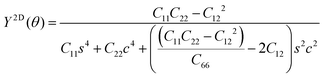(2)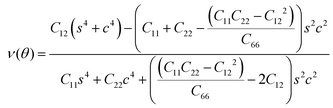(3)
where s = sin(θ) and c = cos(θ), and Y2D(θ) and ν(θ) are the angle dependent Young's modulus and Poisson's ratio, respectively. The 2D polar representation curve in Fig. 3(a) clearly shows that Young's modulus and Poisson's ratio of th-BN are highly anisotropic. As a benchmark, we calculated Young's modulus of h-BN, which was about 267 N m−1, agreeing well with the previous report (271 N m−1).53 Moreover, Young's modulus of t-BN is found to be 218.29 N m−1. For th-BN, the maximum in-plane Young's modulus emerges in the zigzag direction as 232.39 N m−1, whereas the minimum value of ∼175 N m−1 lies in the diagonal direction (or 45°). For the armchair direction, this value is 195.40 N m−1. Thus, the maximum Young's modulus value of th-BN is lower than that of h-BN, however higher than that of t-BN, penta-BN2 (218 N m−1),54 and α-BN-yne (89 N m−1).42 The results indicate that th-BN exhibits a high Young's modulus and strong B3–N3 bonds, and a highly anisotropic mechanical response.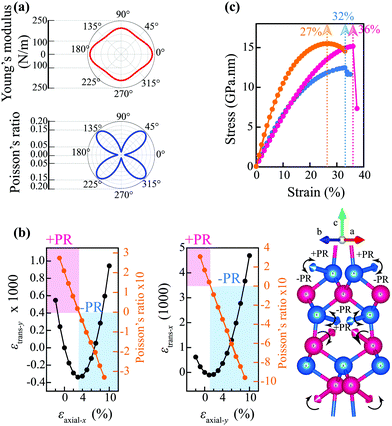Fig. 3 (a) The direction dependence of Young's modulus and Poisson's ratio of th-BN. (b) The variation of response strain in the transverse direction (εtrans) with respect to the applied axial strain (εaxial). The Poisson ratio is embedded to (b), where +PR and −PR represent the positive and negative Poisson ratio regimes, shaded by pink and blue, respectively. The movement direction of atoms in th-BN within the +PR and −PR regions when the structure is subjected to axial strain along the armchair direction. (c) Stress–strain curve of th-BN for zigzag, armchair, and biaxial strains, where the UTS values are depicted in the inset.

Next, we analyze the Poisson's ratio (PR) of th-BN. The PR is defined as the ratio of transverse strain (εtrans) to applied longitudinal strain (εaxial) (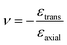). When a positive PR material is stretched along one direction, the material will become thinner along another direction which is perpendicular to the stretching direction. The PR of th-BN is found to be a near-zero positive value of about 0.02 for the zigzag and armchair directions (Fig. 3(a)). The variation of εtrans with respect to εaxial for the zigzag and armchair strains is presented in Fig. 3(b). It is clear that, around the equilibrium state of th-BN, the εtrans value decreases with increasing the εaxial for the zigzag and armchair directions, where it behaves as a positive PR material (non-auxetic). With further strains, however, the variation of εtrans becomes zero at 3% zigzag strain and 1.5% armchair strain, and then simultaneously increases where th-BN behaves as a negative PR material (auxetic). Therefore, the negative PR in th-BN emerges at 3% zigzag and 1.5% armchair strains, respectively. These different threshold values offer the ability to design non-auxetic, auxetic, and partially auxetic (non-auxetic in one direction and auxetic in others) boron nitride. Thus, th-BN exhibits a sign-tunable PR under axial strain. Recently, there has been growing research interest to explore 2D materials exhibiting a tunable Poisson's ratio behavior. Qin et al. have reported the emergence of the negative PR in h-BN at 18% strain.55 Likewise, in our very recent study, we have observed that 2D tetrahex carbon and tetrahex carbides exhibit a sign-tunable PR nature under a very small strain of ∼3%.22 Thus, the emergence of auxetic nature in th-BN under very small strain makes it exceptionally promising for nanomechanical device applications.

To unveil the underlying mechanism of the sign-tunable PR in th-BN, we systematically investigated the variation of the lattice constants governed by bond length, bond angle, and thickness. Such that, as a consequence of response strain, the lattice constants of th-BN in the transverse direction decrease with increasing applied axial strain along the zigzag and armchair directions within the range of positive PR, and then suddenly increases for further strain where the negative PR emerges. The variation of bond length, bond angle, and thickness with respect to zigzag and armchair strains in the range from 0 to 10% is presented in Fig. S4. With the increase of uniaxial strain, the B3–N3, B3–N4, and B4–N3 bonds show an increasing trend in their lengths, which exhibits the auxetic nature of th-BN. On the other hand, the lattice elongation in both directions decreases the thickness of the material, which shows that the out-of-plane PR is positive. The deformation mechanism under uniaxial strain can be explained by the mutual competition between bond lengths, bond angles, and buckling thicknesses. The elongated B–N bond lengths weaken the strength of the bonds, resulting in the decreasing of the C12/C11 ratio. The bond realignment with further strain can be seen with the change of the movement direction of atoms in th-BN within positive PR and negative PR (Fig. 3(b) and Fig. S5). We observed that under a tensile strain applied along the armchair (or zigzag) direction, the major atomic motions are the out-of-plane movement (upward and inward movements) of the B3 and N3 atoms. It can be noted that the movement direction of the B3 atoms suddenly changes to the opposite direction when the strain is larger than 2% along the armchair direction where the negative PR emerges. Therefore, the increase of bond lengths, decrease of thicknesses and especially the variation of dihedral angles, combined together act as the primary factors for the emergence of negative PR.

Ideal strength as an important intrinsic mechanical property is the highest value of stress that a material can withstand without the breaking of atomic bonds. To evaluate the ideal strength of th-BN, we determined the strain–stress relation using a 2 × 2 × 1 supercell. The strain–stress curve for zigzag, armchair, and biaxial tensile strain induced deformations is plotted in Fig. 3(c), where the stress increases linearly with increasing corresponding strains in the harmonic region. Under larger strain, the stress of the material increases non-linearly and ultimately the structure fails. The ultimate strength of th-BN is calculated as 12.47 GPa nm, 15.16 GPa nm, and 15.50 GPa nm with the corresponding to ultimate tensile strain (UTS) limits of 32%, 36%, and 27% along the zigzag, armchair, and biaxial directions, respectively. The calculated UTS values of th-BN are higher than those of h-BN (18%, 26%, and 30% along the zigzag, armchair, and biaxial directions, respectively).56 We attribute this superior strength to the presence of three atomic layers in the th-BN structure. Remarkably, the UTS of th-BN for biaxial strain is also higher than that of t-BN (23%) (Fig. S1) and p-BN (12%)38 even when they have the same atomic layers. Thus, th-BN has ultrahigh ideal strength, outperforming even graphene.57 The extraordinary mechanical properties of th-BN are promising for application in the design of 2D nanomechanical devices.

### 3.4. Electronic properties

Fig. 4(a) shows the electronic band structure of th-BN obtained from the HSE06 hybrid functional. The results indicate that th-BN is a wide band gap semiconductor with a band gap energy of 4.49 eV at the HSE06 level, which is lower than the band gap of h-BN (4.71 eV)14 and t-BN (6.72 eV) (Fig. S1). The conduction band minimum (CBM) of th-BN is located at the Y point of the BZ, while the valence band maximum (VBM) is located between the Γ and X points (slightly offset from the X point), creating an indirect band gap feature. To gain deeper insight into the electronic structure, we plotted the atom- and orbital-projected partial density of states (PDOS) of th-BN, as shown in Fig. 4(b). From the PDOS results, the VBM is mostly determined by the 2pz electrons of the N3 atoms with a minor contribution from 2pz of the B3 atoms, while the CBM originates from the 2pz electrons of the B3 atoms with a minor contribution from 2pz of N3. Thus, the band edges come from the 2pz electrons of the B3 and N3 atoms, which is consistent with the band decomposed charge density of th-BN near the Fermi level (Fig. 4(c)), in a way that, the band decomposed charge density of the VBM is more localized around the N3 atoms, while the charge density of the CBM is mainly localized around the B3 atoms. To illustrate the CBM and VBM energies of th-BN, we aligned the band edge positions (obtained from the HSE06 functional) with respect to the redox potentials of water, as shown in Fig. 4(d). We reveal that the band edge positions of th-BN straddle the water redox potentials, which is just one of the fundamental requirements for a photocatalytic semiconductor. Nevertheless, having a large band gap and the lack of optical absorption in the visible region may limit its application in photocatalytic water-splitting reactions.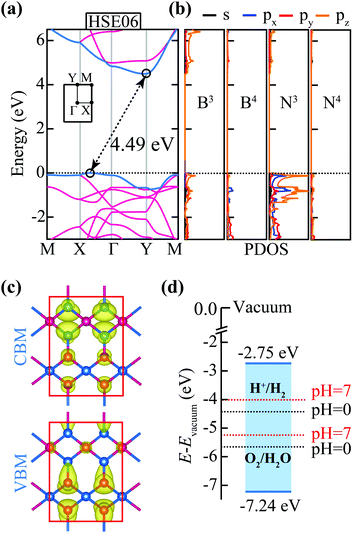Fig. 4 (a) Calculated electronic band structure of th-BN at the HSE06 hybrid functional, where the VBM and CBM are depicted by sky blue lines, and the Fermi energy is set to 0 eV, indicated by the black dashed line. The Brillouin zone is shown as an inset. (b) Calculated atom- and orbital-projected density of states (PDOS) for 3- and 4-fold coordinated boron (B3 and B4) and nitrogen (N3 and N4) atoms at the HSE06 hybrid functional. (c) Band decomposed charge density distributions of the VBM and CBM, where the value for the isosurface is 0.08 e Å−3. (d) Band edge positions (VBM and CBM) of th-BN with respect to the water reduction and oxidation potential levels where the vacuum level is set to 0 eV.

### 3.5. Band gap engineering via strain

Band gap engineering via mechanical strain is an efficient and practical way to modulate the physical and chemical properties of nanomaterials. Previous results have shown that mechanical strain in the tetrahexagonal lattice offers new functionalities such as the transition from a semiconductor to a metal, the band gap transition from indirect to direct, and a pattern change in conductivity.21–24,58 Using the HSE06 hybrid functional, we examined the variation of band gap and band edge positions of th-BN as a function of the zigzag, armchair, and biaxial strains. In the case of the zigzag strain, the position of VBM monotonically increases with increasing strain, whereas that of the CBM decreases, and thereby the band gap energy (governed by the position of VBM and CBM) decreases linearly with increasing strain (Fig. 5(a)). In the case of the armchair strain, the position of VBM and CBM increases linearly with increasing strain within the range from −2 to 10%. The band gap increases linearly with increasing strain up to 4% and then decreases for further tensile strains (Fig. 5(b)). For the biaxial strain, the band gap slightly changes in a non-monotonic manner with respect to isotropic strain (Fig. 5(c)). These results indicate that the strain engineering can effectively modulate the band gap energy and band edge positions of th-BN. It is worth emphasising that although the band gap energy and band edge positions of th-BN are sensitive to the lattice deformations (or strain), the overall electronic band structure is preserved and uniformly (up/down) shifts toward the Fermi level (see Fig. S6). We also note that the band edge positions of th-BN can still straddle the water redox potentials under the corresponding external strains.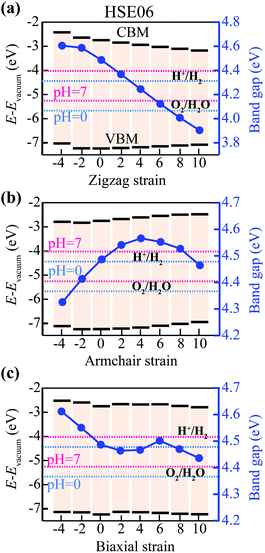Fig. 5 The variation of band edge positions (calculated by the HSE06 functional) of the VBM and CBM of th-BN with respect to the (a) zigzag, (b) armchair, and (c) biaxial strains ranging from −4% (compressive) to 10% (tensile). The sky blue and pink dashed lines represent the water redox potentials at pH = 0 and pH = 7, respectively, to serve as a contrast. The blue solid line and circles indicate the obtained band gaps for the corresponding strains.

### 3.6. Band gap engineering by hydrogenation with an indirect-to-direct band gap transition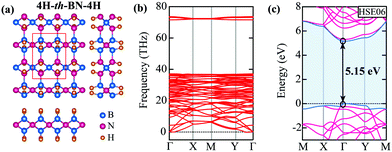Fig. 6 (a) Optimized atomic structure, (b) phonon band dispersions, and (c) the electronic band structure of 4H-th-BN-4H calculated by the HSE06 hybrid functional method. The blue, pink, and orange spheres in (a) represent the boron, nitrogen, and hydrogen atoms, respectively. In (b), the VBM and CBM are depicted by sky blue lines, and the sky blue-shaded area denotes the obtained band gap. The Fermi energy is set to 0 eV indicated by the black dashed line.

### 3.7. Optical properties

To examine the nature of the optical response in th-BN, we calculated the optical absorption coefficient with in-plane light polarization by solving the Bethe–Salpeter equation (BSE) where the quasi-particle (QP) energies and screened interactions W are obtained by GW0 approximation. The optical absorption coefficient α(ω) at an arbitrary frequency ω can be expressed by the following equation: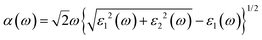(4)
where ε1(ω) and ε2(ω) are the real and imaginary parts of the dielectric function, respectively. Fig. 7 shows the optical absorption coefficient of th-BN as a function of photon energy. We observed that th-BN exhibits strong optical absorption (∼106 cm−1) in the ultraviolet region of the light spectrum. Moreover, the optical response differs significantly for light polarized along the zigzag (x) and armchair (y) directions (i.e. the optical absorption along the armchair direction is much more higher than that along the zigzag direction). The first optical absorption peak in th-BN appears along the zigzag direction at an energy of 5.35 eV, which is in the middle of the ultraviolet range (4.13–6.20 eV). These high optical absorption coefficients and anisotropic optical response features make th-BN very promising for optoelectronic device applications. We further investigated the effect of strain on the optical absorption of th-BN. As shown in Fig. 7, the optical absorption of th-BN exhibits some changes depending on the type of strain. For the zigzag strain, the first absorption peak shifts toward the high energy region under tensile strain, whereas it shifts toward the low energy region under compressive strain. For armchair strain, the prominent peaks slightly shift towards the low energy region under both tension and compression. Hence, the absorption of light with an energy in the middle ultraviolet range can be improved by in-plane strain engineering.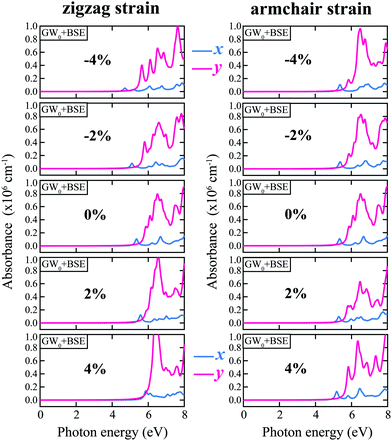Fig. 7 Optical absorption coefficients of th-BN under zigzag and armchair strains from −4% (compressive) to 4% (tensile), where the cyan and pink colors represent the optical absorption for light polarized along the zigzag (x) and armchair (y) directions, respectively.

### 3.8. Bilayer structures

In addition to the th-BN sheet, we also investigated the structural and electronic properties of its bilayer. The bilayer of th-BN can be stacked along the direction perpendicular to its plane in two different configurations, bil1-th-BN and bil2-th-BN, as shown in Fig. 8(a) and (d), respectively. The optimized lattice parameters of bil1-th-BN and bil2-th-BN are listed in Table 1. The calculated interlayer distance of bil1-th-BN and bil2-th-BN structures is 2.31 Å and 1.53 Å, respectively, indicating that the layers in bil1-th-BN are bound to each other by weak van der Waals forces, whereas the layers in bil2-th-BN are covalently bonded. The total energy calculations revealed that bil2-th-BN is more energetically favorable than bil1-th-BN by an energy difference of 0.025 eV per atom. We further examined their dynamic stability by calculating the phonon dispersion curves (Fig. 8(b) and (e)). It can be seen that there are no imaginary phonon modes for bil1-th-BN and bil2-th-BN, confirming their dynamic stability. After verifying their stability, we calculated the electronic band structures and PDOS (Fig. 8(c) and (d)), where bil1-th-BN exhibits a quasi-direct band gap of 3.79 eV, making it promising for optoelectronic device applications. Based on the PDOS results, we found that the main contributions to the band edges come from the pz orbital of the B3 and N3 atoms. Moreover, bil2-th-BN has a large indirect band gap (5.55 eV). The removal of B3 and N3 atoms results in widening of the band gap. Accordingly, it can be seen that in 4H-th-BN-4H, the 3-fold coordinated atoms change to sp3-like ones, leading to the increase of band gap.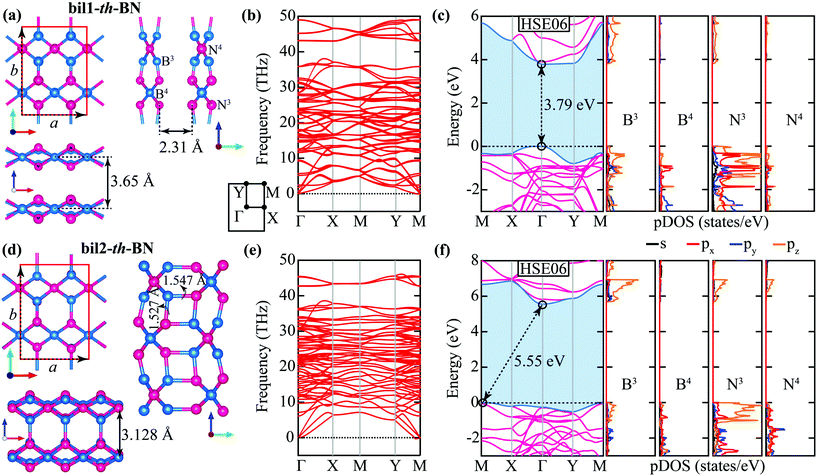Fig. 8 Optimized atomic structures ((a) and (d)), phonon band dispersions ((b) and (e)), and electronic band structures alongside PDOS calculated at the HSE06 hybrid functional level ( (c) and (f)) of bil1-th-BN and bil2-th-BN structures. The blue and pink spheres in (a) and (d) represent the boron and nitrogen atoms, respectively. In (c) and (f), the VBM and CBM are depicted by sky blue lines, and the sky blue-shaded area denotes the obtained band gap. The Fermi energy is set to 0 eV and indicated by the black dashed line.

### 3.9. Suitable substrate for tetrahex carbon

The proposed th-BN sheet has a similar lattice structure to th-C, with lattice mismatch less than 0.5%, indicating that th-BN is ideally suited for heterostructuring with th-C, maintaining its geometrical structure. Therefore, we further examined the structural and electronic properties of the th-C/th-BN heterostructure. The optimized atomic structure of th-C/th-BN is presented in Fig. 9(a). To verify its dynamic stability, we calculated the phonon band dispersions, as shown in Fig. 9(b), where the absence of imaginary frequencies in the phonon dispersions confirms the dynamically stability of the th-C/th-BN heterostructure. We further explore the electronic properties by performing the electronic band structure and PDOS calculations using the HSE06 functional shown in Fig. 9(c). We found that th-C/th-BN exhibits a direct band gap of about 2.22 eV located at the Γ point of the BZ. The main contribution to the PDOS near the Fermi level arises from the sp2-hybridized carbons (C3). Based on the calculated electronic results, we further determined the effective mass of the charge carriers (electrons and holes). The effective mass (m*) is calculated by the parabolic fitting of the Ek curve using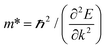, where E is the band-edge energy in the vicinity of the CBM and VBM as a function of wave vector (k). The calculated effective mass of electron (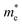) along the Γ–X and Γ–Y edges is about 0.12m0 and 0.58m0, while the hole effective mass is about 3.46m0 and 0.31m0, respectively. We further illustrate the angle dependence of electron and hole effective masses of th-C/th-BN in Fig. 9(d), where it can be seen that th-BN has a highly direction-dependent carrier transport. We further compared the obtained results of the th-C/th-BN heterostructure with those of th-C. We observed that both structural and electronic properties of th-C/th-BN are similar to those of th-C (Fig. 9(e)–(h)). As the interaction between th-C and th-BN in their heterostructures is weak, the th-BN layer in the heterostructure acts as a template with a small lattice mismatch (0.6%), and thus preserves the symmetry and intrinsic electronic properties of th-C. Similar to h-BN as a supporting substrate of graphene (h-C),13–16th-BN can be used as an insulating substrate material for th-C.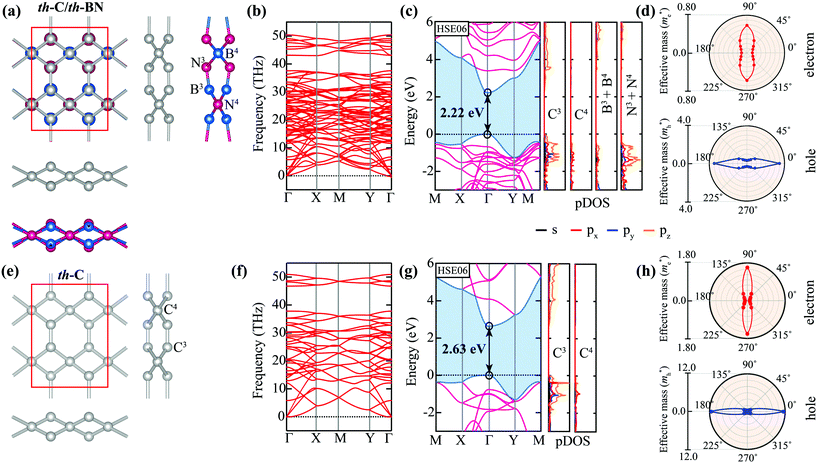Fig. 9 Optimized atomic structures (a) and (e), phonon band dispersions (b) and (f), and electronic band structures alongside PDOS calculated at the HSE06 hybrid functional level (c) and (g), and the angle dependent effective mass of electrons and holes from the Γ–X direction to Γ–A as an arbitrary direction (d) and (h) of the th-C/th-BN and th-C structures. The blue, pink, and silver spheres in (a) and (e) represent the boron, nitrogen, and carbon atoms, respectively. In (c) and (g), the VBM and CBM are depicted by sky blue lines, and the sky blue-shaded area denotes the obtained band gap. The Fermi energy is set to 0 eV and indicated by the black dashed line.

## 4. Conclusion

Based on the first-principles density functional calculations, we successfully predicted a novel 2D boron nitride structure and proved its energetic, dynamic, thermal, and mechanical stability. The intrinsic structural anisotropy of th-BN induces strongly anisotropic mechanical, electronic, and optical behaviors. We found that th-BN has a high in-plane Young's modulus and ultrahigh ideal strength outperforming graphene. Moreover, negative PR in th-BN emerges at very small axial strains (<3%). Thus, th-BN exhibits a sign-tunable Poisson's ratio, offering the ability to become non-auxetic, auxetic, and partially auxetic boron nitride structures. The calculated results indicate that th-BN is a semiconductor with an indirect wide band gap of 4.49 eV. The band gap can be effectively tuned by external strains. Furthermore, the electronic properties of th-BN can be enhanced by hydrogenation. The fully hydrogenated th-BN exhibits an indirect-to-direct band gap transition. th-BN shows high optical absorption in the ultraviolet region. The optical absorption spectrum is strongly direction dependent and tunable by strain, suitable for high performance optoelectronic device applications. th-BN can be stacked into two different configurations, exhibiting exotic electronic properties. The remarkable electronic properties, such as direct band gap and anisotropic effective masses of the th-C/th-BN heterostructure, suggest that th-BN can be used as an insulating substrate for th-C.

## Conflicts of interest

There are no conflicts to declare.

## Acknowledgements

We thank Soheil Ershad Rad and Seymur Jahangirov for fruitful discussions. We also gratefully acknowledge the support from the Brain Pool Program through the National Research Foundation of Korea (NRF) funded by the Ministry of Science and ICT (2020H1D3A1A02081517) and the Nano Materials Research Program through the Ministry of Science and IT Technology under project no. NRF-2016M3A7B4025402.

## References

1. D. Pacile, J. Meyer, Ç. Girit and A. Zettl, Appl. Phys. Lett., 2008, 92, 133107 CrossRef.
2. J. N. Coleman, M. Lotya, A. O'Neill, S. D. Bergin, P. J. King, U. Khan, K. Young, A. Gaucher, S. De and R. J. Smith, Science, 2011, 331, 568–571 CrossRef CAS PubMed.
3. A. K. Geim and I. V. Grigorieva, Nature, 2013, 499, 419 CrossRef CAS PubMed.
4. S. Ipek, M. Kilic, A. Mogulkoc, S. Cahangirov and E. Durgun, Phys. Rev. B, 2018, 98, 241408 CrossRef CAS.
5. R. Pekoz, M. Konuk, M. E. Kilic and E. Durgun, ACS Omega, 2018, 3, 1815–1822 CrossRef CAS PubMed.
6. K. S. Novoselov, A. K. Geim, S. V. Morozov, D. Jiang, Y. Zhang, S. V. Dubonos, I. V. Grigorieva and A. A. Firsov, Science, 2004, 306, 666–669 CrossRef CAS PubMed.
7. X. Du, I. Skachko, A. Barker and E. Y. Andrei, Nat. Nanotechnol., 2008, 3, 491 CrossRef CAS PubMed.
8. C. Lee, X. Wei, J. W. Kysar and J. Hone, Science, 2008, 321, 385–388 CrossRef CAS PubMed.
9. J. H. Seol, I. Jo, A. L. Moore, L. Lindsay, Z. H. Aitken, M. T. Pettes, X. Li, Z. Yao, R. Huang and D. Broido, Science, 2010, 328, 213–216 CrossRef CAS PubMed.
10. A. Nag, K. Raidongia, K. P. Hembram, R. Datta, U. V. Waghmare and C. Rao, ACS Nano, 2010, 4, 1539–1544 CrossRef CAS PubMed.
11. C. Jin, F. Lin, K. Suenaga and S. Iijima, Phys. Rev. Lett., 2009, 102, 195505 CrossRef PubMed.
12. C. Cazorla and T. Gould, Sci. Adv., 2019, 5, eaau5832 CrossRef PubMed.
13. W. Gannett, W. Regan, K. Watanabe, T. Taniguchi, M. Crommie and A. Zettl, Appl. Phys. Lett., 2011, 98, 242105 CrossRef.
14. J. Zhou, Q. Wang, Q. Sun and P. Jena, Phys. Rev. B: Condens. Matter Mater. Phys., 2010, 81, 085442 CrossRef.
15. C. R. Dean, A. F. Young, I. Meric, C. Lee, L. Wang, S. Sorgenfrei, K. Watanabe, T. Taniguchi, P. Kim and K. L. Shepard, Nat. Nanotechnol., 2010, 5, 722 CrossRef CAS PubMed.
16. S. Roth, T. Greber and J. Osterwalder, ACS Nano, 2016, 10, 11187–11195 CrossRef CAS PubMed.
17. S. Demirci, S. E. Rad, S. Kazak, S. Nezir and S. Jahangirov, Phys. Rev. B, 2020, 101, 125408 CrossRef CAS.
18. W. L. Jolly, Modern Inorganic Chemistry, McGraw-Hill College, 1984 Search PubMed.
19. M. Shahrokhi, B. Mortazavi and G. R. Berdiyorov, Solid State Commun., 2017, 253, 51–56 CrossRef CAS.
20. B. Ram and H. Mizuseki, Carbon, 2018, 137, 266–273 CrossRef CAS.
21. M. E. Kilic and K.-R. Lee, Carbon, 2020, 161, 71 CrossRef CAS.
22. M. E. Kilic and K.-R. Lee, Carbon, 2021, 174, 368–381 CrossRef CAS.
23. M. E. Kilic and K.-R. Lee, J. Phys. Chem. C, 2020, 124, 8225–8235 CrossRef CAS.
24. M. E. Kilic and K.-R. Lee, J. Mater. Chem. C, 2021, 9(14), 4971–4977 RSC.
25. P. E. Blöchl, Phys. Rev. B: Condens. Matter Mater. Phys., 1994, 50, 17953 CrossRef PubMed.
26. G. Kresse and D. Joubert, Phys. Rev. B: Condens. Matter Mater. Phys., 1999, 59, 1758 CrossRef CAS.
27. G. Kresse and J. Hafner, J. Phys.: Condens. Matter, 1994, 6, 8245 CrossRef CAS.
28. J. P. Perdew, K. Burke and M. Ernzerhof, Phys. Rev. Lett., 1996, 77, 3865 CrossRef CAS PubMed.
29. H. J. Monkhorst and J. D. Pack, Phys. Rev. B: Solid State, 1976, 13, 5188 CrossRef.
30. L. Chaput, A. Togo, I. Tanaka and G. Hug, Phys. Rev. B: Condens. Matter Mater. Phys., 2011, 84, 094302 CrossRef.
31. S. Nosé, J. Chem. Phys., 1984, 81, 511–519 CrossRef.
32. S. Nosé, Mol. Phys., 1984, 52, 255–268 CrossRef.
33. W. G. Hoover, Phys. Rev. A, 1985, 31, 1695 CrossRef PubMed.
34. J. Heyd, G. E. Scuseria and M. Ernzerhof, J. Chem. Phys., 2003, 118, 8207–8215 CrossRef CAS.
35. J. Paier, M. Marsman, K. Hummer, G. Kresse, I. C. Gerber and J. G. Ángyán, J. Chem. Phys., 2006, 124, 154709 CrossRef CAS PubMed.
36. M. Shishkin and G. Kresse, Phys. Rev. B: Condens. Matter Mater. Phys., 2006, 74, 035101 CrossRef.
37. F. Karlicky and M. Otyepka, J. Chem. Theory Comput., 2013, 9, 4155–4164 CrossRef CAS PubMed.
38. J. Li, X. Fan, Y. Wei and G. Chen, Sci. Rep., 2016, 6, 31840 CrossRef CAS PubMed.
39. M. Topsakal, E. Aktürk and S. Ciraci, Phys. Rev. B: Condens. Matter Mater. Phys., 2009, 79, 115442 CrossRef.
40. W. Tang, E. Sanville and G. Henkelman, J. Phys.: Condens. Matter, 2009, 21, 084204 CrossRef CAS PubMed.
41. A. Savin, R. Nesper, S. Wengert and T. F. Fässler, Angew. Chem., Int. Ed. Engl., 1997, 36, 1808–1832 CrossRef CAS.
42. V. O. Ozcelik and S. Ciraci, J. Phys. Chem. C, 2013, 117, 2175–2182 CrossRef CAS.
43. G. Li, Y. Li, H. Liu, Y. Guo, Y. Li and D. Zhu, ChemComm, 2010, 46, 3256–3258 RSC.
44. A. Kara, H. Enriquez, A. P. Seitsonen, L. L. Y. Voon, S. Vizzini, B. Aufray and H. Oughaddou, Surf. Sci. Rep., 2012, 67, 1–18 CrossRef CAS.
45. A. K. Singh, K. Mathew, H. L. Zhuang and R. G. Hennig, J. Phys. Chem. Lett., 2015, 6, 1087–1098 CrossRef CAS PubMed.
46. A. K. Singh, H. L. Zhuang and R. G. Hennig, Phys. Rev. B: Condens. Matter Mater. Phys., 2014, 89, 245431 CrossRef.
47. C. He, L. Sun, C. Zhang, X. Peng, K. Zhang and J. Zhong, Phys. Chem. Chem. Phys., 2012, 14, 10967–10971 RSC.
48. S. Cahangirov, M. Topsakal, E. Aktürk, H. Şahin and S. Ciraci, Phys. Rev. Lett., 2009, 102, 236804 CrossRef CAS PubMed.
49. H. Tornatzky, R. Gillen, H. Uchiyama and J. Maultzsch, Phys. Rev. B, 2019, 99, 144309 CrossRef CAS.
50. G. Qin, Q.-B. Yan, Z. Qin, S.-Y. Yue, M. Hu and G. Su, Phys. Chem. Chem. Phys., 2015, 17, 4854–4858 RSC.
51. M. Born and K. Huang, Dynamical Theory of Crystal Lattices, Oxford, 1954 Search PubMed.
52. E. Cadelano, P. L. Palla, S. Giordano and L. Colombo, Phys. Rev. B: Condens. Matter Mater. Phys., 2010, 82, 235414 CrossRef.
53. K. N. Kudin, G. E. Scuseria and B. I. Yakobson, Phys. Rev. B: Condens. Matter Mater. Phys., 2001, 64, 235406 CrossRef.
54. T. Zhang, Y. Ma, B. Huang and Y. Dai, ACS Appl. Mater. Interfaces, 2019, 11, 6104–6110 CrossRef CAS PubMed.
55. G. Qin and Z. Qin, npj Comput. Mater., 2020, 6, 1–6 CrossRef.
56. Q. Peng, W. Ji and S. De, Comput. Mater. Sci., 2012, 56, 11–17 CrossRef CAS.
57. F. Liu, P. Ming and J. Li, Phys. Rev. B: Condens. Matter Mater. Phys., 2007, 76, 064120 CrossRef.
58. X. Peng, Q. Wei and G. Yang, Carbon, 2020, 165, 37–44 CrossRef CAS.
59. Y. Wang, Phys. Status Solidi RRL, 2010, 4, 34–36 CrossRef CAS.
60. F. Karlickỳ, R. Zbořil and M. Otyepka, J. Chem. Phys., 2012, 137, 034709 CrossRef PubMed.

### Footnote

Electronic supplementary information (ESI) available: Optimized atomic configurations, phonon band dispersion, electronic band structure, strain energy vs. strain and stress–strain curve of t-BN; optimized atomic configurations and phonon band dispersion of h-BN; electron localization function of th-BN, and variation of bond length, bond angle, and buckling thickness of th-BN; moving atom directions of th-BN under axial strain; electronic band structure of th-BN; and atomic configurations and adsorption energy of the hydrogenated derivatives of th-BN. See DOI: 10.1039/d1nr00734c
 This journal is © The Royal Society of Chemistry 2021